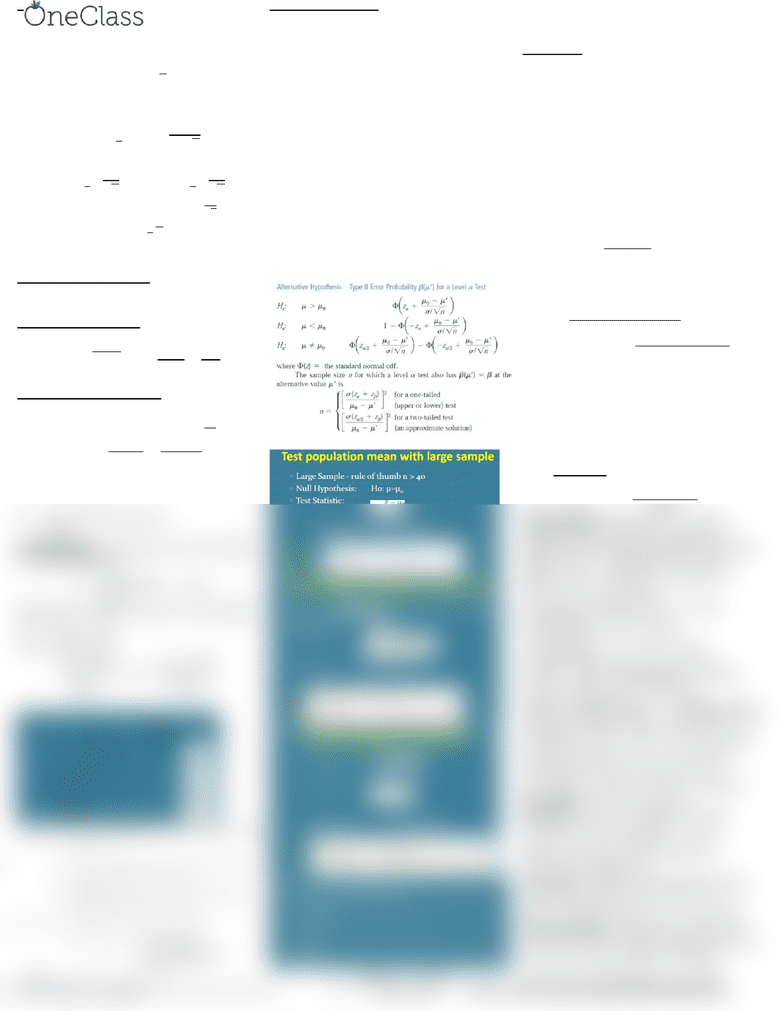Study Guides (400,000)
US (230,000)
U of M (5,000)
IOE (10)
Final

# IOE 265 Study Guide - Final Guide: Standard Deviation, Covariance, Central Limit Theorem

Department
Industrial And Operations Engineering
Course Code
IOE 265
Professor
Xiuli Chao
Study Guide
Final

This preview shows half of the first page. to view the full 2 pages of the document.Confidence Intervals: Identify a plausible
range for point estimate
Ex. 95% confidence range implies 95% chance
that true value is in range
Find multiplier with invNorm(,0,1)
Use sample std. dev of
Note: Sum of independent normal random
variables leads to   and   
 
  
 
For probability  we have:
    
For one-sided CI (u):   
For all mean CI:   
Note: round-up, width is ½ of interval
Must know variance + normal dist.
Central Limit Theorem: When n is large
enough (, distribution of sample mean is
approx. normal (  )
Proportion Estimation: Bernoulli:
  
CI:     

Note: For n, use p and q as .5 to be safe
Chi-Squared Distribution: If population has
normal distribution then:
has normal dist. Mean Variance
Sample Variance 

is Chi-
Square Distributed with n-1 df
If Z is standard normal, and
is Chi-Square
dist. with n degrees freedom:
 is t-dist with n df
T-Distribution: Area under curve to the right
of t critical value is
 

Norm w/out mean and variance, small sample
CI:  

CI for variance


 


Hypothesis Testing: Make a claim
Reject or fail to reject that claim
Null hypothesis: 
Alternate hypothesis: 
1: Fix level of significance, acceptable prob of
rejecting null when it’s true
2: Compute prob. of observing sample, take z-
value and turn into p-value
3: If p-value is smaller than sig. level, reject
null hypo, otherwise fail to reject
You need:
Null and alternative hypothesis
Test statistic what decision is on
Rejection region (when to reject)
Significance level : probability, given
hypothesis is correct, of rejecting it
Type II error is Beta, fail to reject + H0 false
Reject the null if p-value < alpha (smaller p-
value is stronger evidence against H0)
Mu0 is actual and mu’ is test


Note: smallest level of signiﬁcance at which
you would be willing to reject the null
hypothesis is the P-value.
Definitions: Probability measure of
likelihood for something to occur. Statistics
concerned with learning from data. Types:
categorial (qualitative) numerical (quantitative)
Univariate: observations on 1 variable
Bivariate: observations on 2 variables
Discrete: Finite possible values
Continuous: any real value in interval
Dot plot: each datapoint is dot on a line
Stem&Leaf: First part of each piece of data on
left hand (stem) side. Remaining digits on
right-hand (leaf) side in order. Add a key
Frequency Analysis: # of frequencies of each
class/value in data set (relative, cumulative)
# of ranges is , 5-20
Histogram is graphical rep. for 1 variable
(exponential, normal, bi-modal, skewed)
Graph of rel. freq w/ width 1 is samp density
Density: 

 
Median: middle obs., further from skew
Trimmed mean: Eliminate % of values from
both ends, re-compute mean
Fourth spread (IQR): Upper 4th Lower 4th
Outlier is 1.5*IQR from closest 4th, Extreme
Outlier is 3*IQR away (open circle)
Mode: most common, Range: max-min

 sample uses N-1


Part 2 Mod 1: Sample space: set of all
possible outcomes. Events: any subset of
sample space. Probability: likelihood of event
A\B is 

N1 possible outcomes then N2 = N1*N2
Permutation (w/order):

Combination (w/out order):

P(A|B)
 Independence:
 

Discrete RV (pmf): 
Cumulative:    

Expected value: 
E(ax+b)=aE(x) +b, V(ax+b) = a2V(x)
V(x =   
Bernoulli: E(x) = p, V(x)=p(1-p)
Binomial: Bin(n,p): n=number of trials,
p=prob of success is

Multiple Bernoulli becomes binomial
E(x) = np, V(x)=np(1-p)
Geometric: Number of independent B trials
until success p(x)=(1-p)x-1p, E(x) = 1/p,
V(x) = (1-p)/p2, P(    
Hypergeometric: Batch has N products, M
good and N-M bad, pick N products, prob of x
good: h(x:n,M,N) =


E(x) = np, V(x)=
 , p=
find more resources at oneclass.com
find more resources at oneclass.com